Area Under a CurveCustom SearchAREA UNDER A CURVE To find the area under a curve, we must agree on what is desired. In figure 6-1, where f(x) is equal to the constant 4 and the "curve" is the straight line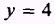the area of the rectangle is found by multiplying the height times the width. Thus, the area under the curve is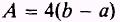The next problem is to find a method for deterŁmining the area under any curve, provided that the curve is continuous. In figure 6-2, the area under the curve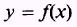between points x and x +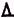x is approximately. We consider thatx is small and the area given isA .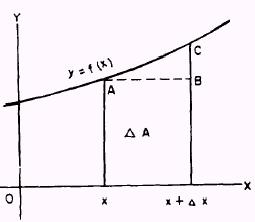Figure 6-2.-AreaA. This area under the curve is nearly a rectangle. The areaA, under the curve, would differ from the area of the rectangle by the area of the triangle ABC if AC were a straight line. Whenx becomes smaller and smaller, the area of ABC becomes smaller at a faster rate, and ABC finally becomes indistinguishable from a triangle. The area of this triangle becomes negligible whenx is sufficiently small.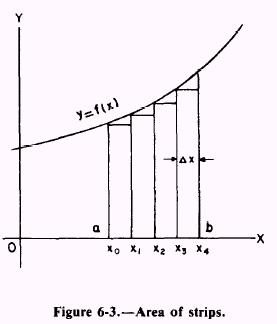Therefore, for sufficiently small values ofx, we can say thatNow, if we have the curve in figure 6-3, the sum of all the rectangles will be approximately equal to the area under the curve and bounded by the lines at a and b. The difference between the actual area under the curve and the sum of the areas of the recŁtangles will be the sum of the areas of the triangles above each rectangle. Asx is made smaller and smaller, the sum of the rectangular areas will approach the value of the area under the curve. The sum of the areas of the rectangles may be indicated by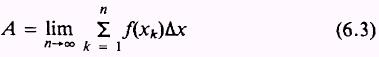where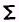(sigma) is the symbol for sum, n is the number of recŁtangles,is the area of each rectangle, and k is the designaŁtion number of each rectangle. In the particular example just discussed, where we have four rectangles, we would write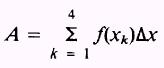and we would have only the sum of four rectangles and not the limiting area under the curve. When using the limit of a sum, as in equation (6.3), we are required to use extensive algebraic techniques to find the actual area under the curve. To this point we have been given a choice of using arithmetic and finding only an approximation of the area under a curve or using extensive algebra to find the actual area. We will now use calculus to find the area under a curve fairly easily. In figure 6-4, the areas under the curve, from a to b, is shown as the sum of the areas of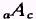and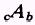. The notation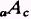, means the area under the curve from a to c. The Intermediate Value Theorem states thatwhere f(c) in figure 6-4 is the value of the function at an intermediate point between a and b.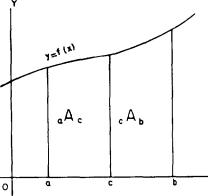Figure 6-4.-Designation of limits. We now modify figure 6-4 as shown in figure 6-5. When x=a thenWe see in figure 6-5 that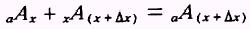therefore, the increase in area, as shown, is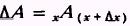Figure 6-5.-Increments of area at fc).   Reference to figure 6-5 shows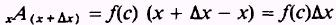where c is a point between a and b. Then by substitution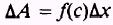or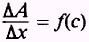and as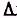x approaches zero, we haveNow, from the definition of integrationwhere C is the constant of integration, andbuttherefore,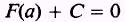By solving for C, we haveand by substituting -F(a) into equation (6.4), we findIf we let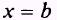thenwhere F(b) and F(a) are the integrals of the function of the curve at the values b and a. The constant of integration C is omitted in equation (6.5) because when the function of the curve at b and a is integrated, C will occur with both F(a) and F(b) and will therefore be subŁtracted from itself. NOTE: The concept of the constant of integration is more fully explained later in this chapter. EXAMPLE. Find the area under the curve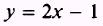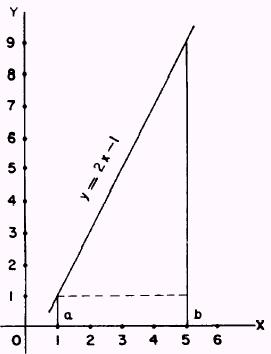Figure 6-6.-Area of triangle and rectangle. in figure 6-6, bounded by the vertical lines at a and b and the X axis. SOLUTION. We know that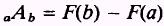and we find thatThen, substituting the values for a and b into, we find that whenand when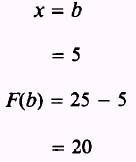Then by substituting these values inwe find thatWe may verify this by considering figure 6-6 to be a triangle with base 4 and height 8 sitting on a rectangle of height 1 and base 4. By known formulas, we find the area under the curve to be 20.Integrated Publishing, Inc. - A (SDVOSB) Service Disabled Veteran Owned Small Business